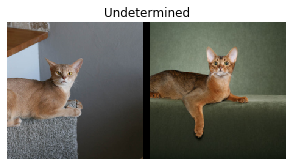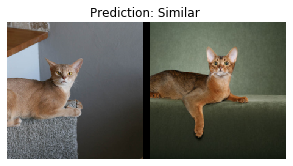# 24_tutorial.siamese.ipynb — monkey-patch `siampredict` method to `Learner`?

For the Siamese network tutorial (24_tutorial.siamese.ipynb, fastai2 links here and here), to make it easier to look at prediction results, I monkey-patched a `siampredict` method to the `Learner`, so that when a `SiameseImage` is passed to it, it will also show the two images and the resulting prediction of “Similar” or “Not similar”.

``````@patch
def siampredict(self:Learner, item, rm_type_tfms=None, with_input=False):
res = self.predict(item, rm_type_tfms=None, with_input=False)
if res == tensor(0):
SiameseImage(item, item, 'Prediction: Not similar').show()
else:
SiameseImage(item, item, 'Prediction: Similar').show()
return res
``````

I also modified the `SiameseImage.show` method so that for a `SiameseImage` that was created without a “label” (i.e. without the 3rd argument of True/False or “similar”/“not similar”), when `show()` is called it will still show the two images, with title “Undetermined”.

``````class SiameseImage(Tuple):
def show(self, ctx=None, **kwargs):
if len(self) > 2:
img1,img2,similarity = self
else:
img1,img2 = self
similarity = 'Undetermined'
if not isinstance(img1, Tensor):
if img2.size != img1.size: img2 = img2.resize(img1.size)
t1,t2 = tensor(img1),tensor(img2)
t1,t2 = t1.permute(2,0,1),t2.permute(2,0,1)
else: t1,t2 = img1,img2
line = t1.new_zeros(t1.shape, t1.shape, 10)
return show_image(torch.cat([t1,line,t2], dim=2), title=similarity, ctx=ctx, **kwargs)
``````

With these two changes, in the tutorial when I want to use a trained model to do a quick inference on a test image (in combination with a reference image), I can do the following:

``````imgref = PILImage.create(reffilepath)
imgtest = PILImage.create(testfilepath)
siamtest = SiameseImage(imgref, imgtest)
siamtest.show();
````````````learn.siampredict(siamtest);
``````I am not sure if this is the correct way to patch things in a notebook, and/or whether these changes are useful for the tutorial notebook or not. Comments welcome : )

Yijin

5 Likes

Can we make a confusion matrix from learner in Siamese tutorial.

Is there any way to visualise the difference or similarity activation map using gradcam in saimese network.